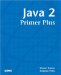# Increment and Decrement OperatorsJava™ 2 Primer PlusBy Steven Haines, Steve Potts Table of Contents Chapter 3.  Operators

 In computer programming it is quite common to want to increase or decrease the value of an integer type by 1. Because of this Java provides the increment and decrement operators that add 1 to a variable and subtract 1 from a variable, respectively. The increment operator is denoted by two plus signs (++), and the decrement operator is denoted by two minus signs (--). The form of the increment and decrement operators is ` variable++; ++variable; variable--; --variable; ` For example: ` int i = 10; i++; // New value of i is 11 ` You might notice that the variable could either be prefixed or suffixed by the increment or decrement operator. If the variable is always modified appropriately (either incremented by 1 or decremented by 1), then what is the difference? The difference has to do with the value of the variable that is returned for use in an operation. Prefixing a variable with the increment or decrement operator performs the increment or decrement, and then returns the value to be used in an operation. For example: ` int i = 10; int a = ++i; // Value of both i and a is 11 i = 10; int b = 5 + --i; // Value of b is 14 (5 + 9) and i is 9 ` Suffixing a variable with the increment or decrement operator returns the value to be used in the operation, and then performs the increment or decrement. For example: ` // Value of i is 11, but the value of a is 10 // Note that the assignment preceded the increment int i = 10; int a = i++; // Value of i is 9 as before, but the value of b is 15 (5 + 10) i = 10; int b = 5 + i--; ` Pay particular attention to your code when you use prefix and postfix versions of these operators and be sure that you completely understand the difference. That difference has led many programmers on a search for unexplained behavior in their testing!TopJava 2 Primer Plus
ISBN: 0672324156
EAN: 2147483647
Year: 2001
Pages: 332

Similar book on Amazon